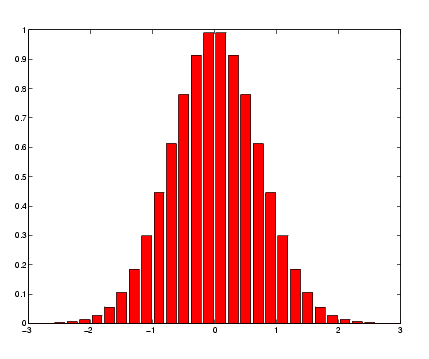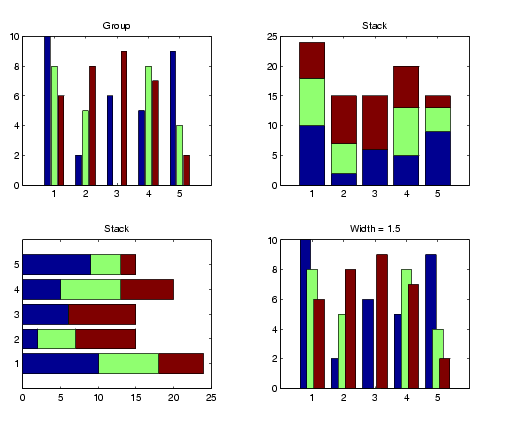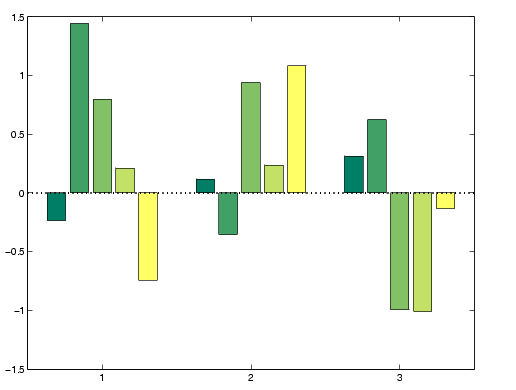MATLAB Function Referencebar, barh

Plot bar graph (vertical and horizontal)

Syntax

• ```bar(Y)
bar(x,Y)
bar(...,width)
bar(...,'`style`')
bar(...,`'``bar_color``'`)
bar(axes_handle,...)
h = bar(...)
hpatches = bar('v6',...)

barh(...)
h = barh(...)
hpatches = barh('v6',...)
```

Description

A bar graph displays the values in a vector or matrix as horizontal or vertical bars.

```bar(Y) ``` draws one bar for each element in `Y`. If `Y` is a matrix, `bar` groups the bars produced by the elements in each row. The x-axis scale ranges from 1 up to `length(Y)` when `Y` is a vector, and `1` to `size(Y,1)`, which is the number of rows, when `Y` is a matrix. The default is to scale the x-axis to the highest x-tick on the plot, (a multiple of 10, 100, etc.). If you want the x-axis scale to end exactly at the last bar, you can use the default, and then, for example, type

• ```set(gca,'xlim',[1 length(Y)])
```

at the MATLAB prompt.

```bar(x,Y) ``` draws a bar for each element in `Y` at locations specified in `x`, where `x` is a vector defining the x-axis intervals for the vertical bars. The x-values can be nonmonotonic, but cannot contain duplicate values. If `Y` is a matrix, `bar` groups the elements of each row in `Y` at corresponding locations in `x`.

```bar(...,width) ``` sets the relative bar width and controls the separation of bars within a group. The default `width` is `0.8`, so if you do not specify `x`, the bars within a group have a slight separation. If `width` is `1`, the bars within a group touch one another.

```bar(...,'style') ``` specifies the style of the bars. `'``style``'` is `'grouped'` or `'stacked'`. `'group'` is the default mode of display.

• `'grouped'` displays m groups of n vertical bars, where m is the number of rows and n is the number of columns in `Y`. The group contains one bar per column in `Y`.
• `'stacked'` displays one bar for each row in `Y`. The bar height is the sum of the elements in the row. Each bar is multicolored, with colors corresponding to distinct elements and showing the relative contribution each row element makes to the total sum.

```bar(...,'bar_color') ``` displays all bars using the color specified by the single-letter abbreviation `'r'`, `'g'`, `'b'`, `'c'`, `'m'`, `'y'`, `'k'`, or `'w'`.

```bar(axes_handles,...) and barh(axes_handles,...) ``` plot into the axes with handle `axes_handle` instead of the current axes (`gca`).

```h = bar(...) ``` returns a vector of handles to barseries graphics objects. `bar` creates one barseries graphics object per column in `Y`.

```barh(...) and h = barh(...) ``` create horizontal bars. `Y` determines the bar length. The vector `x` is a vector defining the y-axis intervals for horizontal bars. The x-values can be nonmonotonic, but cannot contain duplicate values.

Backward Compatible Versions

```hpatches = bar('v6',...) and hpatches = barh('v6',...) ``` return the handles of patch objects instead of barseries objects for compatibility with MATLAB 6.5 and earlier. See patch object properties for a discussion of the properties you can set to control the appearance of these bar graphs.

See Plot Objects and Backward Compatibility for more information.

Barseries Objects

Creating a bar graph of an m-by-n matrix creates m groups of n barseries objects. Each barseries object contains the data for corresponding `x` values of each bar group (as indicated by the coloring of the bars).

Note that some barseries object properties set on an individual barseries object set the values for all barseries objects in the graph. See the property descriptions for information on specific properties.

Examples

Single Series of Data

This example plots a bell-shaped curve as a bar graph and sets the colors of the bars to red.

• ```x = -2.9:0.2:2.9;
bar(x,exp(-x.`*`x),'r')```

Bar Graph Options

This example illustrates some bar graph options.

• ```Y = round(rand(5,3)`*`10);
subplot(2,2,1)
bar(Y,'group')
title 'Group'
subplot(2,2,2)
bar(Y,'stack')
title 'Stack'
subplot(2,2,3)
barh(Y,'stack')
title 'Stack'
subplot(2,2,4)
bar(Y,1.5)
title 'Width = 1.5'```

Setting Properties with Multiobject Graphs

This example creates a graph that displays three groups of bars and contains five barseries objects. Since all barseries objects in a graph share the same baseline, you can set values using any barseries object's `BaseLine` property. This example uses the first handle returned in `h`.

• ```Y = randn(3,5);
h = bar(Y);
set(get(h(1),'BaseLine'),'LineWidth',2,'LineStyle',':')
colormap summer ```% Change the color scheme
``````

See Also

`bar3`, `ColorSpec`, `patch`, `stairs`, `hist`

Area, Bar, and Pie Plots for related functions

Bar and Area Graphs for more examplesbalance bar3, bar3h© 1994-2005 The MathWorks, Inc.